Following with the previous article, I tested further a few more cases to benchmark the runtime performance on various JIT compilation libraries (Numba, JAX, TensorFlow and PyTorch). In this time, I am going to test with a more realistic example used in machine learning and numerical computation - linear regression.

## Linear regression

Linear regression basically models the response (dependent variable) and the explanatory variables (independent variables) in a linear relationship.

There are already lots of approaches to derive the weights and bias from the model. I will employ the gradient descent approach, which includes matrix multiplication and iteration. The approach and numerical operations per se are the core of modern machine learning and optimization models.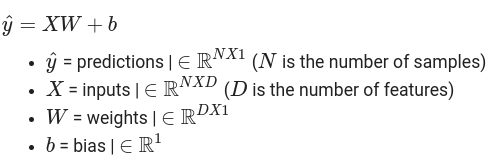First, the loss function is defined as the mean squared error (MSE)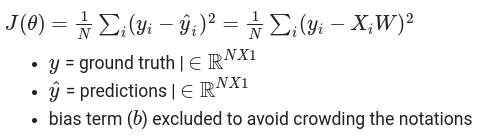The gradients of the loss function can be derived as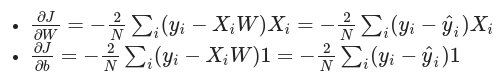and the solution is iterated with the gradients and learning rate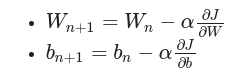## Training data

The training data is generated by a linear model with adding normalized noises. The length of the training data is always 10k, while the dimension of the model varies from 2 to 100. The objective is to emulate the real world usage of fitting the model with various numbers of features.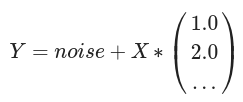## Implementation

We will quickly go through each implementation and compare the ease of adapting the library from rewriting the Python function to switching between CPU and GPU. The following libraries are compared

• Python / NumPy (CPU only)

• Numba (CPU only, despite its GPU support)

• JAX (CPU and GPU)

• Tensorflow (CPU and GPU)

• PyTorch (CPU and GPU)

## Python / NumPy

First, beating NumPy performance is not trivial as lots of linear algebra operations have been optimized to pass down into low level library OpenBLAS (or Intel MKL if supported in the platform). It means not only the functions are written in C implementation already but also NumPy can leverage on the continuous development in C / FORTRAN written numerical libraries.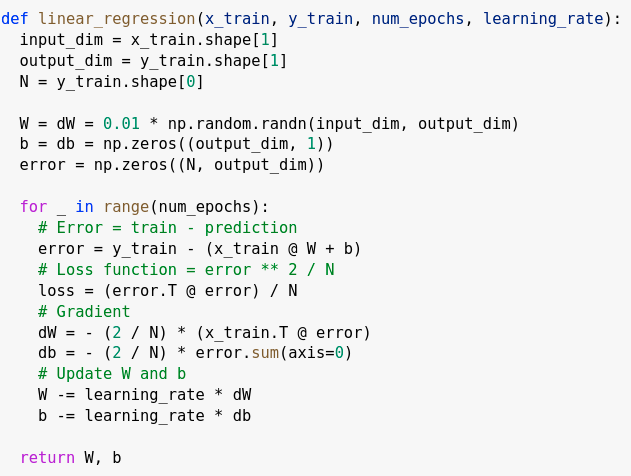Basically the implementation is to first compute the error by the estimator W and b, and then the gradients, update the new estimation of W and b by the learning rate and the gradients, and finally iterate the process for a specified number of times. In real world, the process should stop when the estimator converges or the total error is smaller than a specified tolerance level. But here, to ensure each iteration are completed in the same number of iterations, no early stopping condition is implemented.

For GPU implementation, some external libraries, like CuPy, keep most of the NumPy function signatures / prototypes same to ease the pain migrating to switch from CPU to GPU. But it is out of the scope today to compare with it as it is not in the NumPy supported scope.

## Numba

As mentioned in the previous article, to compile the Python function in Numba is straightforward - just put the jit function decorator on top of the function. But now, as the matrix / array operations are involved, specifying the contiguous type of the NumPy array inputs can give deterministic result. For example, Numba can give you hints (actually warnings) when you multiply the two opposite contiguous matrices are multiplied.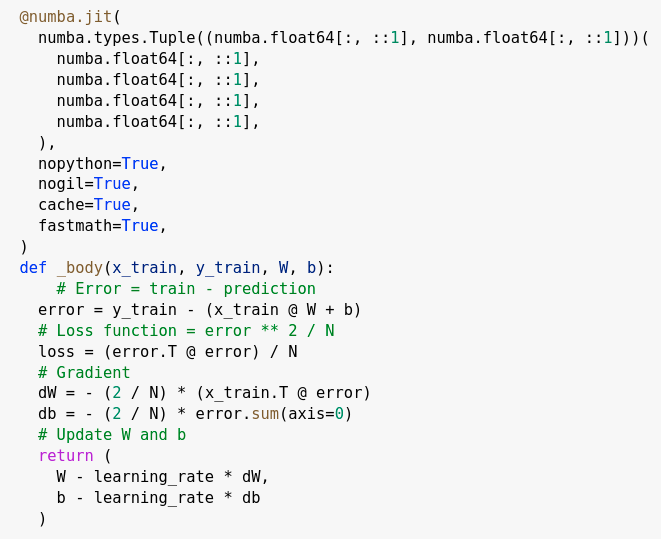Though Numba supports CUDA GPU, the GPU implementation requires rewriting the function, like handling kernel invocation and thread positioning. It is definitely not an easy switch from CPU to GPU under Numba, so we are not going to compare with Numba GPU part here.

## JAX

The “numpy” module in JAX keeps most of the NumPy functions and their signatures, so switching from Numpy function to JAX can be hugely benefited.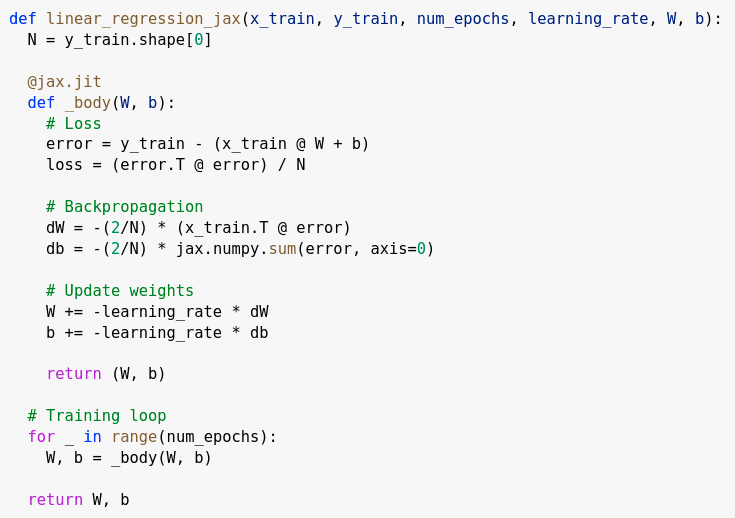JAX uses XLA (domain-specific compiler for linear algebra developed by TensorFlow) to compile and can run the function both in CPU and GPU seamlessly. The function can be switched to run in GPU in background when JAX refers to the GPU devices in the platform during import.

The GPU compatibility is one of the major focuses in JAX development team, and they leverage on asynchronous dispatch to speed up the critical path from mapping the array memory from CPU to GPU. It is a big bonus when you have platforms in both CPU and GPU and can easily switch between them, for example in Colab supporting both devices.

## TensorFlow

Though TensorFlow does not keep the same NumPy function prototypes in its linear algebra module, TensorFlow operators have been well documented and designed in a good sense to use. Also lots of researchers and ML developers already have the TensorFlow usage / experience. Migrating the NumPy operators to the TensorFlow ones is not entirely cumbersome.

To ensure the function is compiled in optimal paths, we just need to wrap the function with TensorFlow function decorator and then enable the JIT in it.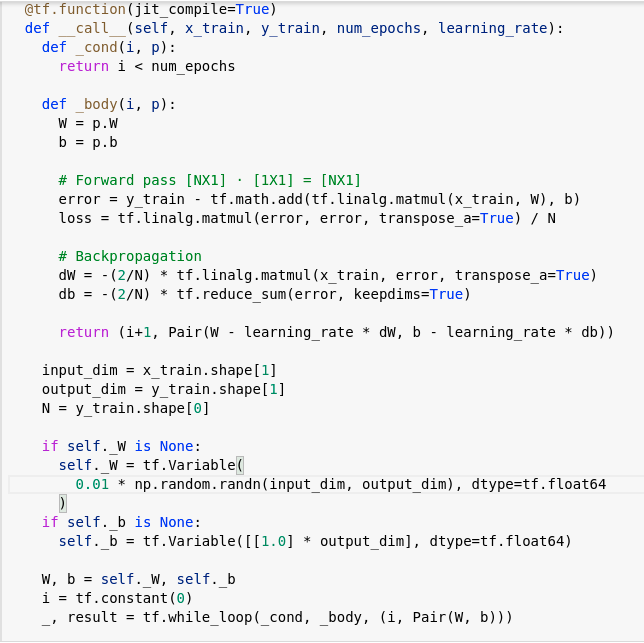Again, TensorFlow uses XLA to compile and optimize the critical paths. Same as JAX, all the operators and models can be seamlessly switched between CPU and GPU.

(Similar to JAX, actually TensorFlow now has a NumPy module, but it is still in experimental stage, and it may induce overhead in dispatching, so the implementation was not written in its NumPy module)

## PyTorch

PyTorch has been catching up a lot to TensorFlow in recent years and performs much better on a lot of models and areas. Same as most ML frameworks, CPU and GPU targets are both supported and can easily be transferred to each other.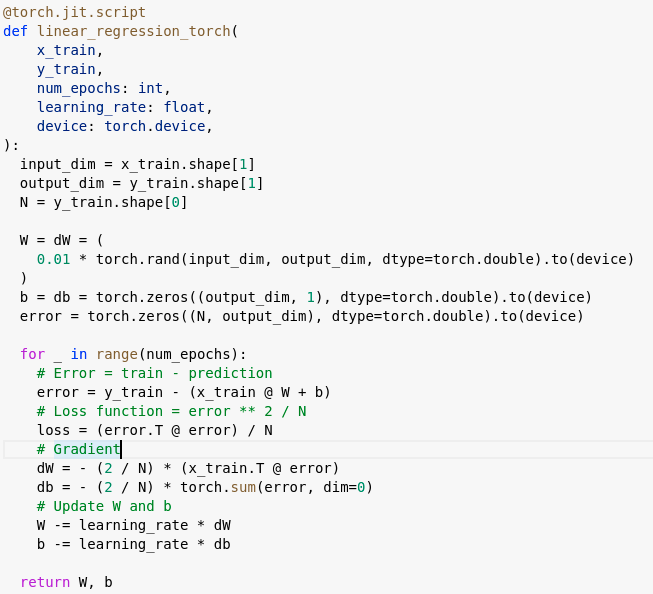## Performance

The benchmark is run with a range of dimensions in the example training data, from 2 to 100. For example, in a dimension of 100, the training data is in size of 10k x 100 (around 8 Mb in float64 type).

In the following table, the green shaded part is the CPU compiled runtime, while the blue shaded part is the GPU one.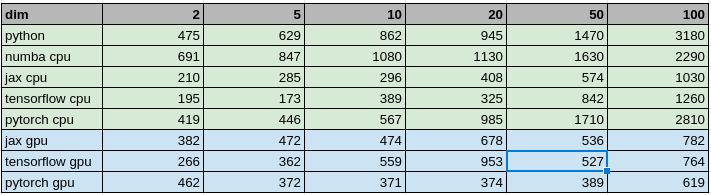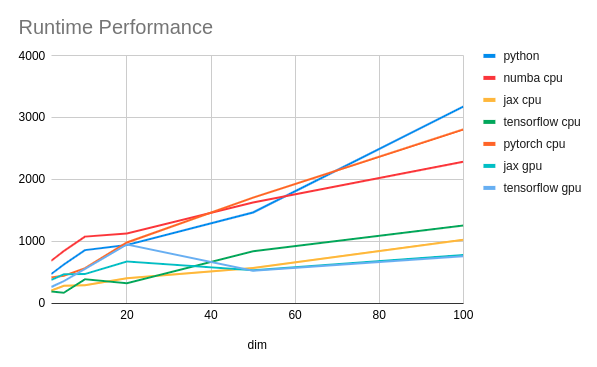### CPU

We can see that in CPU the winner is JAX while TensorFlow does not fall apart too much. Both achieve in 2-3 times improvement in runtime. I am staggered to see Numba does not perform better than primitive NumPy implementation, until meeting the vast dimension of the model.

### GPU

All the GPU implementations runs much faster than the NumPy one. The highest one is 5 times faster. PyTorch performs slightly better in high dimension than the other two XLA compiled implementations.

## Conclusion

In terms of the runtime performance, development effort, and the compatibility of GPU devices, all the three prevailing machine learning / deep learning oriented libraries, i.e. JAX, TensorFlow and PyTorch, are ahead of NumPy and Numba. Thanks to the huge contribution from the ML industry, these libraries provide amazing framework for scientific and numerical related researches in boosting the Python performance.

All these framework also draws a clear benchmark for future Python numerical libraries - if the library cannot easily support running on multiple devices (e.g. now Google doubles its bets on TPU), it will be quickly out of the game.

## Reference / Acknowledgment

All the source codes can be found in the Colab notebook. Special thanks to Cyril Chimisov of his advice on JAX and TensorFlow part.